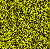The puzzlehunt has ended, thanks for playing!

# Colonel Mustard## Solution

author: Nic Bricknell

We are presented with an image, 50 pixels wide and 49 pixels high, with yellowish colours. We can thus interpret the title, 'Colonel Mustard', in that the colours are 'mustard' and represent a 49x50 matrix for which we need to find the kernel, a 50-vector. Given that the matrix has integral components, and the kernel is unique up to a multiplicative constant, we then scale the kernel to the simplest integer vector. Labelling 1 as A, 2 as B, etc, we can then find the message, `iliketothinkoysterstranscendnationalbarriers`.

Example solution code:

```-- Convert image to an intensity matrix, find its null vector (kernel),
-- scale such that components are simple integers and try 1=a, 2=b, etc..
-- Output is iliketothinkoysterstranscendnationalbarriers.

-- This example solution has been tested with:
-- * GHC 7.10.3
-- * JuicyPixels 3.2.7.1
-- * HMatrix 0.17.0.2

import System.IO( openFile, IOMode( ReadMode ) )
import Data.ByteString( hGetContents )
import Data.Char( chr )
import Data.Function( (&) )
import Numeric.LinearAlgebra(
Matrix, Vector, Z, R, build, fromZ, null1, toList )
import Codec.Picture.Bitmap( decodeBitmap )
import Codec.Picture.Types(
Image( .. ), DynamicImage( .. ), PixelRGB8( .. ), Pixel8, pixelAt )

main :: IO ()
main = do
fileContents <- hGetContents =<< openFile "./colonelmustard.bmp" ReadMode
case decodeBitmap fileContents of
Right im -> im & intensityMatrix & decode & putStrLn

intensityMatrix :: DynamicImage -> Matrix Z
intensityMatrix (ImageRGB8 im@(Image w h _)) = build (h, w) intensityAt
where
intensityAt :: Z -> Z -> Z -- HMatrix lib forces us to use this signature.
intensityAt i j = sum \$ fromIntegral <\$> [r, g, b]
where
PixelRGB8 r g b = pixelAt im x y where [y, x] = fromEnum <\$> [i, j]

decode :: Matrix Z -> String
decode m =
m & (fromZ :: Matrix Z -> Matrix R) -- (Need a matrix of reals to do SVD.)
& (null1 :: Matrix R -> Vector R) -- Get the kernel of the matrix.
& (toList :: Vector R -> [Double]) -- (Vector has no Functor instance.)
&
-> (flip (/) \$ v !! 0) <\$> v -- Scale such that (v !! 0) == 1.0.
& (fmap round :: [Double] -> [Int])
& drop 5 -- Throw away timing pattern [1, 2, 3, 4, 5].
& init -- Throw away large negative integer at the end.
& fmap ((+) 96) & fmap chr -- 1=a, 2=b, etc..
```